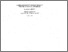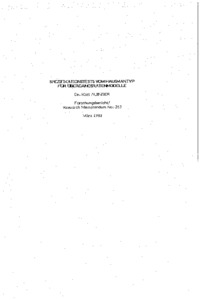# spezifikationstests vom hausmantyp fuer uebergangsratenmodelle

Auinger, Karl (March 1988) spezifikationstests vom hausmantyp fuer uebergangsratenmodelle. Closed Series > Forschungsberichte / Research Memoranda 243Preview
Text
fo243.pdf

Download (3MB) | Preview

## Abstract or Table of Contents

summary: in this paper we construct different tests against misspecification for the basic failure time model with a constant hazard function. throughout the paper it is taken into account that lifetime data are usually censored. we consider the following censoring mechanisms: (i) single type i-censoring (ii) type ii-censoring (iii) random censoring using a functional equation of the survival function ((s(x+y)=s(x)(y)), we treat the cases (i) and (iii) by the following method: for fixed x,y we estimate the value s(x+y) in two different ways such that the (standardized) difference between these estimates is asymptotically normal. using a consistent estimate of the asymptotic variance, we derive test-statistics that are - under the null-hypothesis - asymptotically chi-square. for the case (ii) we use order statistics to estimate different quantiles. then the ratio of two of these estimates is (when appropriately standardized) asymptotically normal with known variance. finally, for the case (i) we propose a further method: estimating the scale parameter by maximum-likelihood method once using the given censoring time c, once using an "artificial" censoring time c'<c, we obtain two estimates whose standardized difference is asymptotically normal. for all these possibilities we determine the sets of alternativesagainst which the tests are consistent.;

Item Type: IHS Series Published 26 Sep 2014 10:34 01 Apr 2016 14:07 https://irihs.ihs.ac.at/id/eprint/243

### Actions (login required)View Item